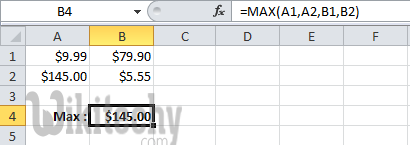# pig tutorial - apache pig tutorial - Apache Pig - MAX() Function - pig latin - apache pig - pig hadoop

## What is Max() function?

• MAX() function is used to calculate the highest value for a column in a single-column bag
• The Max() function will ignores the NULL values while calculating the maximum value
• The Max() function will return the maximum value in a set of values.
• MAX() requires a preceding GROUP ALL statement for global maximums and a GROUP BY statement for group maximums.
• The MAX() function is a built-in function which can be called as Statistical Function.Learn apache pig - apache pig tutorial - max function in apache pig - apache pig examples - apache pig programs

## Example

wikitechy_employee_details.txt

• We have loaded this file into Pig with the relation name called wikitechy_employee_details which is given below:

## Calculating the Maximum GPA

• We need to group the relation wikitechy_employee_details using the Group All operator, and we need to store the result in the relation called employee_group_all which is given below:

This will produce a relation for calculating the maximum GPA as shown below.

• Now, we will calculate the global maximum of GPA, of all the employees which is done using the MAX() function which is shown below.